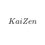# Two Red Lines in a Rectangle

Imagine a rectangle with sides $$\overline{\text{AB}}$$, $$\overline{\text{BC}}$$, $$\overline{\text{CD}}$$, and $$\overline{\text{DA}}$$. Side $$\overline{\text{AB}}$$ has a length of $$4$$ while $$\overline{\text{BC}}$$ has a length of $$6$$.

Put point $\text{E}$ on $\overline{\text{AD}}$. Draw a line from $\text{E}$ to $\text{B}$ and draw another line from $\text{E}$ to $\text{C}$.

If $\angle\text{BEC}$ has a measurement of $65°$, what is the length of line segments $\overline{\text{EB}}$ and $\overline{\text{EC}}$?Rectangle $\text{ABCD}$

How can I solve for the lengths of the red lines? A step-by-step solution will be very much appreciated.

Here's the link of an image of described shape: http://imgur.com/gallery/x6kGdAqNote by Kaizen Cyrus
1 year ago

This discussion board is a place to discuss our Daily Challenges and the math and science related to those challenges. Explanations are more than just a solution — they should explain the steps and thinking strategies that you used to obtain the solution. Comments should further the discussion of math and science.

When posting on Brilliant:

• Use the emojis to react to an explanation, whether you're congratulating a job well done , or just really confused .
• Ask specific questions about the challenge or the steps in somebody's explanation. Well-posed questions can add a lot to the discussion, but posting "I don't understand!" doesn't help anyone.
• Try to contribute something new to the discussion, whether it is an extension, generalization or other idea related to the challenge.
• Stay on topic — we're all here to learn more about math and science, not to hear about your favorite get-rich-quick scheme or current world events.

MarkdownAppears as
*italics* or _italics_ italics
**bold** or __bold__ bold
- bulleted- list
• bulleted
• list
1. numbered2. list
1. numbered
2. list
Note: you must add a full line of space before and after lists for them to show up correctly
paragraph 1paragraph 2

paragraph 1

paragraph 2

[example link](https://brilliant.org)example link
> This is a quote
This is a quote
    # I indented these lines
# 4 spaces, and now they show
# up as a code block.

print "hello world"
# I indented these lines
# 4 spaces, and now they show
# up as a code block.

print "hello world"
MathAppears as
Remember to wrap math in $$ ... $$ or $ ... $ to ensure proper formatting.
2 \times 3 $2 \times 3$
2^{34} $2^{34}$
a_{i-1} $a_{i-1}$
\frac{2}{3} $\frac{2}{3}$
\sqrt{2} $\sqrt{2}$
\sum_{i=1}^3 $\sum_{i=1}^3$
\sin \theta $\sin \theta$
\boxed{123} $\boxed{123}$

## Comments

Sort by:

Top Newest

Here's a hint: let $\overline{DE}$ be $x$. Then, $\overline{AE} = 6-x$. We can use the Pythagorean Theorem to express $\overline{EB}$ and $\overline{EC}$ in terms of $x$. Then, you can use Cosine Law on $\triangle EBC$ and solve for $x$. Plug the result back into $\overline{EB}$ and $\overline{EC}$, and the problem is solved!

- 1 year ago

Log in to reply

$\overline{AE}$ is equal to $6-x$? But $\overline{AD}$ is $6$ and you said let it be $x$.

- 1 year ago

Log in to reply

Whoops, my mistake. I meant let $\overline{DE}$ be $x$. Everything else stays the same.

- 1 year ago

Log in to reply

×

Problem Loading...

Note Loading...

Set Loading...